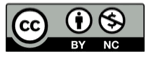# 圆的公式和图像

2018-06-25## 定义

• 直角坐标：$(x-a)^2+(y-b)^2=R^2$
• 参数方程：$[x(t), y(t)]=[a+R\cos(t),b+R\sin(t)]$

## 捐助本站